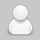2019
01-21

# Turtlebot咖啡机-设置客户最佳的角度

```from tf import transformations
import math```

```#90 degrees.  You can this specify this to whatever your customer wants
turtlebot_orientation_in_degrees = 90
#convert euler to quaternion and save in new variable quat
quat = transformations.quaternion_from_euler(0, 0, math.radians(turtlebot_orientation_in_degrees))
#pass quaternion values to pose
goal.target_pose.pose = Pose(Point(-0.73, -0.9, 0.000), Quaternion(quat, quat, quat, quat))```